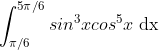# Basic maths-calculus-Q5

+1 vote

The value of the integralis ____?asked May 19
reshown May 24

We know the following property of definite integral :For the given question,

(because  )

Let the given intergral equation be L, then we have L = -L;

2L = 0

L = 0answered May 27 by (112,390 points)